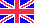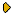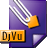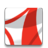Журнал Экспериментальной и Теоретической Физики
 НАЧАЛО | ПОИСК | ДЛЯ АВТОРОВ | ПОМОЩЬОбщая информация о журналеЗолотые страницыАдреса редакцииСодержание журналаСообщения редакцииПравила для авторовЗагрузить статьюПроверить статус статьиЖЭТФ, Том 130, Вып. 5, стр. 796 (Ноябрь 2006)
(Английский перевод - JETP, Vol. 103, No 5, p. 690, November 2006 доступен on-line на www.springer.com )

WAVE FUNCTIONS OF HELIUM-LIKE SYSTEMS IN LIMITING REGIONS

Поступила в редакцию: 12 Июня 2006

PACS: 32.80.Fb, 31.15.JaDJVU (89.8K)PDF (302.8K)

We find an approximate analytic forms for the solutions Ψ(r1, r2, r12) of the Schrodinger equation for a system of two electrons bound to a nucleus in the spatial regions r1=r2=0 and r12=0 that are of great importance for a number of physical processes. The forms are based on the well-known behavior of Ψ(r1, r2, r12) near the singular triple coalescence point. The approximate functions are compared to the locally precise ones obtained earlier by the correlation function hyperspherical harmonic (CFHH) method for the helium atom, light helium-like ions, and the negative ion of hydrogen H-. The functions are shown to determine a natural basis for the expansion of CFHH functions in the considered spatial region. We demonstrate how these approximate functions simplify calculations of high-energy ionization processes.

Сообщить о технических проблемах